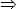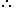UPSC  >  Unit Digit Calculation - Practice Test

# Unit Digit Calculation - Practice Test

Test Description

## 5 Questions MCQ Test UPSC Prelims Paper 2 CSAT - Quant, Verbal & Decision Making | Unit Digit Calculation - Practice Test

Unit Digit Calculation - Practice Test for UPSC 2022 is part of UPSC Prelims Paper 2 CSAT - Quant, Verbal & Decision Making preparation. The Unit Digit Calculation - Practice Test questions and answers have been prepared according to the UPSC exam syllabus.The Unit Digit Calculation - Practice Test MCQs are made for UPSC 2022 Exam. Find important definitions, questions, notes, meanings, examples, exercises, MCQs and online tests for Unit Digit Calculation - Practice Test below.
Solutions of Unit Digit Calculation - Practice Test questions in English are available as part of our UPSC Prelims Paper 2 CSAT - Quant, Verbal & Decision Making for UPSC & Unit Digit Calculation - Practice Test solutions in Hindi for UPSC Prelims Paper 2 CSAT - Quant, Verbal & Decision Making course. Download more important topics, notes, lectures and mock test series for UPSC Exam by signing up for free. Attempt Unit Digit Calculation - Practice Test | 5 questions in 10 minutes | Mock test for UPSC preparation | Free important questions MCQ to study UPSC Prelims Paper 2 CSAT - Quant, Verbal & Decision Making for UPSC Exam | Download free PDF with solutions
 1 Crore+ students have signed up on EduRev. Have you?
Unit Digit Calculation - Practice Test - Question 1

### What is the unit digit in the product (365 x 659 x 771) ?

Detailed Solution for Unit Digit Calculation - Practice Test - Question 1

Explanation:

Unit digit in 34 = 1Unit digit in (34)16 = 1Unit digit in 365 = Unit digit in [ (34)16 x 3 ] = (1 x 3) = 3

Unit digit in 659 = 6

Unit digit in 74Unit digit in (74)17 is 1.

Unit digit in 771 = Unit digit in [(74)17 x 73] = (1 x 3) = 3Required digit = Unit digit in (3 x 6 x 3) = 4.

Unit Digit Calculation - Practice Test - Question 2

### What is the unit digit in 7105 ?

Detailed Solution for Unit Digit Calculation - Practice Test - Question 2

Explanation:

Unit digit in 7105 = Unit digit in [ (74)26 x 7 ]

But, unit digit in (74)26 = 1Unit digit in 7105 = (1 x 7) = 7

Unit Digit Calculation - Practice Test - Question 3

### The unit digit in the product (784 x 618 x 917 x 463) is:

Detailed Solution for Unit Digit Calculation - Practice Test - Question 3

Unit digit in the given product = Unit digit in (4 x 8 x 7 x 3) = (672) = 2

Unit Digit Calculation - Practice Test - Question 4

The unit digit of the sum 1289 + 2541 + 8215 + 6137 is:

Detailed Solution for Unit Digit Calculation - Practice Test - Question 4

Solution: The required answer is the unit digit of the sum of the unit digits of the numbers in given sum. i.e., The unit digit of (1289 + 2541 + 8215 + 6137) = the unit digit of (9+1+5+7) = the unit digit of (22) = 2 Hence the answer is 2.

Unit Digit Calculation - Practice Test - Question 5

The unit digit of the product 1022 x 729 x 889 x 971 is:

Detailed Solution for Unit Digit Calculation - Practice Test - Question 5

a)2

Solution:

The required answer is the unit digit of the product of the unit digits of the numbers in given product.
i.e, The unit digit of (1022 x 729 x 889 x 971)
= the unit digit of (2 x 9 x 9 x 1)
= the unit digit of (162)
= 2

Hence, the answer is 2.

## UPSC Prelims Paper 2 CSAT - Quant, Verbal & Decision Making

67 videos|50 docs|151 tests
 Use Code STAYHOME200 and get INR 200 additional OFF Use Coupon Code
Information about Unit Digit Calculation - Practice Test Page
In this test you can find the Exam questions for Unit Digit Calculation - Practice Test solved & explained in the simplest way possible. Besides giving Questions and answers for Unit Digit Calculation - Practice Test, EduRev gives you an ample number of Online tests for practice

## UPSC Prelims Paper 2 CSAT - Quant, Verbal & Decision Making

67 videos|50 docs|151 tests

### How to Prepare for UPSC

Read our guide to prepare for UPSC which is created by Toppers & the best Teachers